## 2016年5月23日 星期一

### ASP.NET MVC Elmah Dashboard - 查看 Elmah 存在 SQL Server 的記錄

ASP.NET MVC + ELMAH 監控並記錄你的網站錯誤資訊 1 基本安裝與介紹
ASP.NET MVC + ELMAH 監控並記錄你的網站錯誤資訊 2 修改 elmah 的預設瀏覽路徑
ASP.NET MVC + ELMAH 監控並記錄你的網站錯誤資訊 3 elmah 的信件通知設定與 favicon.icon 404 錯誤過濾
ASP.NET MVC + ELMAH 監控並記錄你的網站錯誤資訊 4 將 elmah 錯誤記錄儲存到 MS SQL Server 中
ELMAH - 自訂錯誤通知郵件的設定(於特定Exception)
ASP.NET MVC 使用Glimpse監測網站的一舉一動 3 - 結合ELMAH
ASP.NET MVC 3 使用 ELMAH 無法記錄正確 Http Status Code ? （此篇已收錄於 elmah 官網 Wiki - WebBase 單元中 http://code.google.com/p/elmah/wiki/WebBase
ASP.NET MVC 使用 Elmah 但不安裝 Elmah.MVC 的 location 修改
Elmah.MVC 2.0.0
ELMAH.MVC 2.0.1 - 可以自訂瀏覽路徑
ELMAH - 設定錯誤通知信件的主旨
ASP.NET MVC 與 Elmah.MVC 2.1.1 以及使用 Windows Azure SQL Database 的Schema 修改

https://github.com/twMVC/twMVC-2-2

### ASP.NET MVC Elmah Dashboard

http://www.arebis.be/MvcElmahDashboard/

https://www.nuget.org/packages/Arebis.Web.Mvc.ElmahDashboard/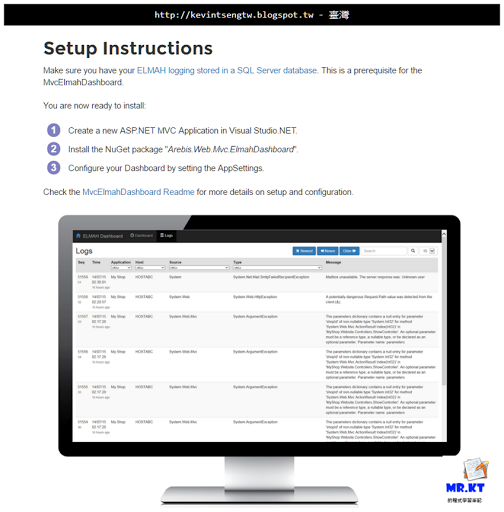MVC Elmah Dashboard 首頁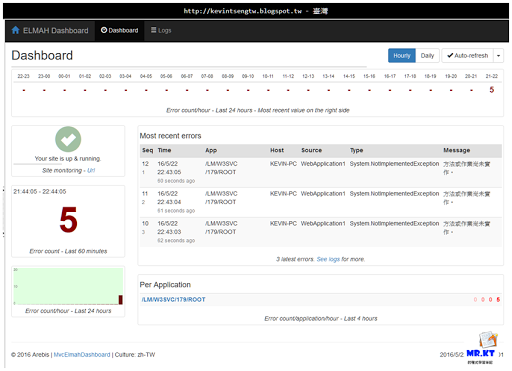Logs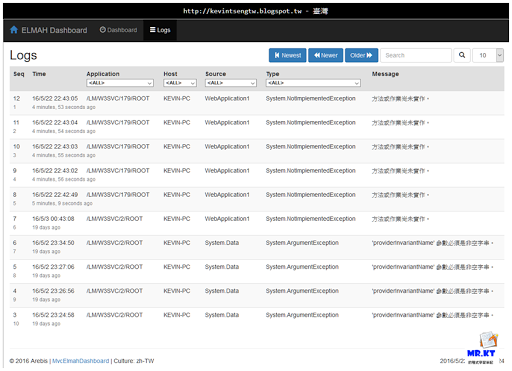Log Details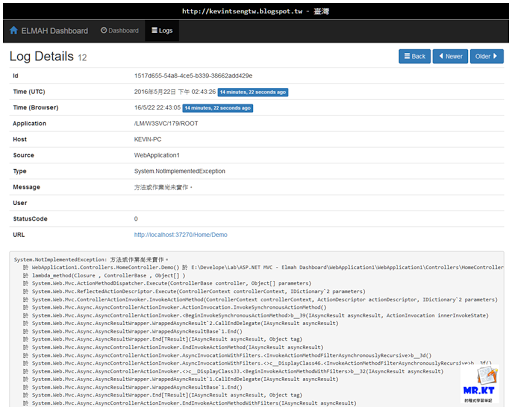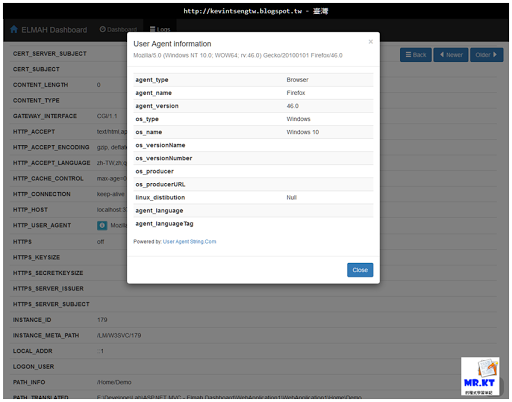Log 查詢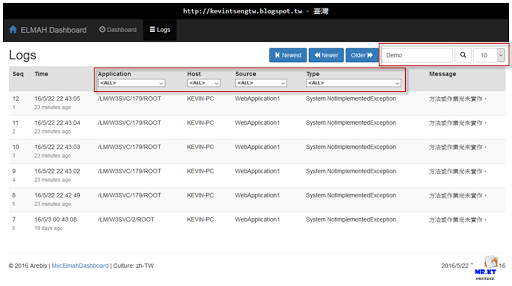### 安裝說明

Step.1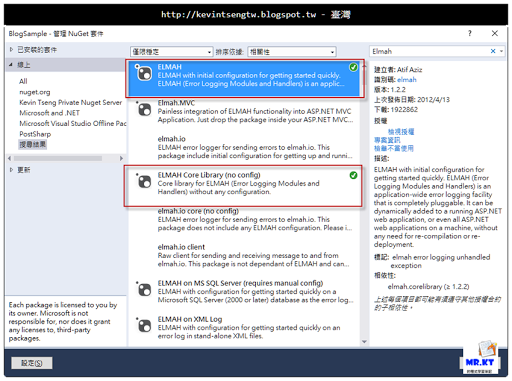Step.2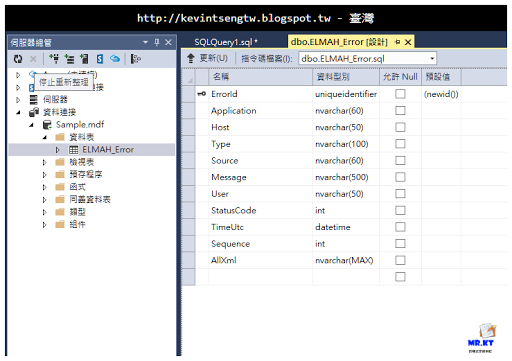[ELMAH.Error] Table Create Schema

`--~Changing index [dbo].[ELMAH_Error].PK_ELMAH_Error to a clustered index.  You may    want to pick a different index to cluster on.`
`SET ANSI_NULLS ON`
`SET QUOTED_IDENTIFIER ON`
`IF NOT EXISTS (SELECT * FROM sys.objects WHERE object_id = OBJECT_ID(N'[dbo].    [ELMAH_Error]') AND type in (N'U'))`
`BEGIN`
`CREATE TABLE [dbo].[ELMAH_Error](`
`[ErrorId] [uniqueidentifier] NOT NULL,`
`[Application] [nvarchar](60) NOT NULL,`
`[Host] [nvarchar](50) NOT NULL,`
`[Type] [nvarchar](100) NOT NULL,`
`[Source] [nvarchar](60) NOT NULL,`
`[Message] [nvarchar](500) NOT NULL,`
`[User] [nvarchar](50) NOT NULL,`
`[StatusCode] [int] NOT NULL,`
`[TimeUtc] [datetime] NOT NULL,`
`[Sequence] [int] IDENTITY(1,1) NOT NULL,`
`[AllXml] [nvarchar](max) NOT NULL,`
`CONSTRAINT [PK_ELMAH_Error] PRIMARY KEY CLUSTERED `
`(`
`[ErrorId] ASC`
`)WITH (STATISTICS_NORECOMPUTE  = OFF, IGNORE_DUP_KEY = OFF)`
`)`
`END`
` `
`IF NOT EXISTS (SELECT * FROM sys.indexes WHERE object_id = OBJECT_ID(N'[dbo].[ELMAH_Error]') AND name = N'IX_ELMAH_Error_App_Time_Seq')`
`CREATE NONCLUSTERED INDEX [IX_ELMAH_Error_App_Time_Seq] ON [dbo].[ELMAH_Error] `
`(`
`[Application] ASC,`
`[TimeUtc] DESC,`
`[Sequence] DESC`
`)WITH (STATISTICS_NORECOMPUTE  = OFF, IGNORE_DUP_KEY = OFF, DROP_EXISTING = OFF, ONLINE  = OFF)`
`GO`
`IF NOT EXISTS (SELECT * FROM dbo.sysobjects WHERE id =     OBJECT_ID(N'[DF_ELMAH_Error_ErrorId]') AND type = 'D')`
`BEGIN`
`ALTER TABLE [dbo].[ELMAH_Error] ADD  CONSTRAINT [DF_ELMAH_Error_ErrorId]  DEFAULT (newid()) FOR [ErrorId]`
`END`
` `
`GO`
`SET ANSI_NULLS ON`
`SET QUOTED_IDENTIFIER ON`
`IF NOT EXISTS (SELECT * FROM sys.objects WHERE object_id = OBJECT_ID(N'[dbo].[ELMAH_GetErrorsXml]') AND type in (N'P', N'PC'))`
`BEGIN`
`EXEC dbo.sp_executesql @statement = N'`
`CREATE PROCEDURE [dbo].[ELMAH_GetErrorsXml]`
`(`
`@Application NVARCHAR(60),`
`@PageIndex INT = 0,`
`@PageSize INT = 15,`
`@TotalCount INT OUTPUT`
`)`
`AS `
` `
`SET NOCOUNT ON`
` `
`DECLARE @FirstTimeUTC DATETIME`
`DECLARE @FirstSequence INT`
`DECLARE @StartRow INT`
`DECLARE @StartRowIndex INT`
` `
`SELECT `
`    @TotalCount = COUNT(1) `
`FROM `
`    [ELMAH_Error]`
`WHERE `
`    [Application] = @Application`
` `
`-- Get the ID of the first error for the requested page`
` `
`SET @StartRowIndex = @PageIndex * @PageSize + 1`
` `
`IF @StartRowIndex <= @TotalCount`
`BEGIN`
` `
`    SET ROWCOUNT @StartRowIndex`
` `
`    SELECT  `
`        @FirstTimeUTC = [TimeUtc],`
`        @FirstSequence = [Sequence]`
`    FROM `
`        [ELMAH_Error]`
`    WHERE   `
`        [Application] = @Application`
`    ORDER BY `
`        [TimeUtc] DESC, `
`        [Sequence] DESC`
` `
`END`
`ELSE`
`BEGIN`
` `
`    SET @PageSize = 0`
` `
`END`
` `
`-- Now set the row count to the requested page size and get`
`-- all records below it for the pertaining application.`
` `
`SET ROWCOUNT @PageSize`
` `
`SELECT `
`    errorId     = [ErrorId], `
`    application = [Application],`
`    host        = [Host], `
`    type        = [Type],`
`    source      = [Source],`
`    message     = [Message],`
`    [user]      = [User],`
`    statusCode  = [StatusCode], `
`    time        = CONVERT(VARCHAR(50), [TimeUtc], 126) + ''Z''`
`FROM `
`    [ELMAH_Error] error`
`WHERE`
`    [Application] = @Application`
`AND`
`    [TimeUtc] <= @FirstTimeUTC`
`AND `
`    [Sequence] <= @FirstSequence`
`ORDER BY`
`    [TimeUtc] DESC, `
`    [Sequence] DESC`
`FOR`
`    XML AUTO`
` `
`' `
`END`
`GO`
`SET ANSI_NULLS ON`
`SET QUOTED_IDENTIFIER ON`
`IF NOT EXISTS (SELECT * FROM sys.objects WHERE object_id = OBJECT_ID(N'[dbo].[ELMAH_GetErrorXml]') AND type in (N'P', N'PC'))`
`BEGIN`
`EXEC dbo.sp_executesql @statement = N'`
`CREATE PROCEDURE [dbo].[ELMAH_GetErrorXml]`
`(`
`@Application NVARCHAR(60),`
`@ErrorId UNIQUEIDENTIFIER`
`)`
`AS`
` `
`SET NOCOUNT ON`
` `
`SELECT `
`    [AllXml]`
`FROM `
`    [ELMAH_Error]`
`WHERE`
`    [ErrorId] = @ErrorId`
`AND`
`    [Application] = @Application`
` `
`' `
`END`
`GO`
`SET ANSI_NULLS ON`
`SET QUOTED_IDENTIFIER ON`
`IF NOT EXISTS (SELECT * FROM sys.objects WHERE object_id = OBJECT_ID(N'[dbo].    [ELMAH_LogError]') AND type in (N'P', N'PC'))`
`BEGIN`
`EXEC dbo.sp_executesql @statement = N'`
`CREATE PROCEDURE [dbo].[ELMAH_LogError]`
`(`
`@ErrorId UNIQUEIDENTIFIER,`
`@Application NVARCHAR(60),`
`@Host NVARCHAR(30),`
`@Type NVARCHAR(100),`
`@Source NVARCHAR(60),`
`@Message NVARCHAR(500),`
`@User NVARCHAR(50),`
`@AllXml NVARCHAR(MAX),`
`@StatusCode INT,`
`@TimeUtc DATETIME`
`)`
`AS`
` `
`SET NOCOUNT ON`
` `
`INSERT`
`INTO`
`    [ELMAH_Error]`
`    (`
`        [ErrorId],`
`        [Application],`
`        [Host],`
`        [Type],`
`        [Source],`
`        [Message],`
`        [User],`
`        [AllXml],`
`        [StatusCode],`
`        [TimeUtc]`
`    )`
`VALUES`
`    (`
`        @ErrorId,`
`        @Application,`
`        @Host,`
`        @Type,`
`        @Source,`
`        @Message,`
`        @User,`
`        @AllXml,`
`        @StatusCode,`
`        @TimeUtc`
`    )`
` `
`' `
`END`
`GO`

`CREATE NONCLUSTERED INDEX [IX_ELMAH_Error_Application] ON [dbo].[ELMAH_Error] ([Application] ASC)`
`GO`
`CREATE NONCLUSTERED INDEX [IX_ELMAH_Error_Host] ON [dbo].[ELMAH_Error] ([Host] ASC)`
`GO`
`CREATE NONCLUSTERED INDEX [IX_ELMAH_Error_Type] ON [dbo].[ELMAH_Error] ([Type] ASC)`
`GO`
`CREATE NONCLUSTERED INDEX [IX_ELMAH_Error_Source] ON [dbo].[ELMAH_Error] ([Source] ASC)`
`GO`
`CREATE NONCLUSTERED INDEX [IX_ELMAH_Error_User] ON [dbo].[ELMAH_Error] ([User] ASC)`
`GO`
`CREATE NONCLUSTERED INDEX [IX_ELMAH_Error_TimeUtc] ON [dbo].[ELMAH_Error] ([TimeUtc] ASC)`
`GO`
`CREATE NONCLUSTERED INDEX [IX_ELMAH_Error_Sequence] ON [dbo].[ELMAH_Error] ([Sequence] ASC)`
`GO`

Step.3

`<;connectionStrings>`
`    <;add name="Elmah.Sql"`
`         connectionString="Data Source=(LocalDB)\MSSQLLocalDB;AttachDbFileName=|DataDirectory|\Sample.mdf;Integrated Security=True"`
`         providerName="System.Data.SqlClient" />;`
`</connectionStrings>    `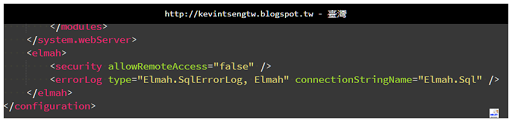Step.4

https://www.nuget.org/packages/Arebis.Web.Mvc.ElmahDashboard/

PM > Install-Package Arebis.Web.Mvc.ElmahDashboard

or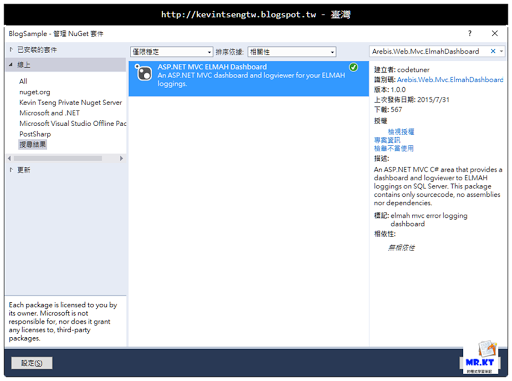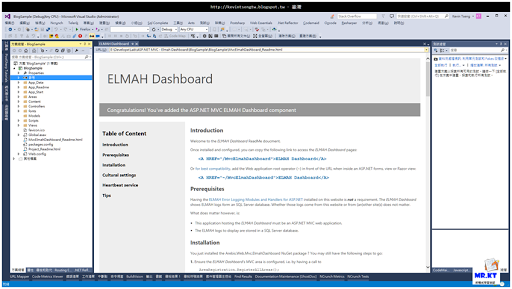Step.5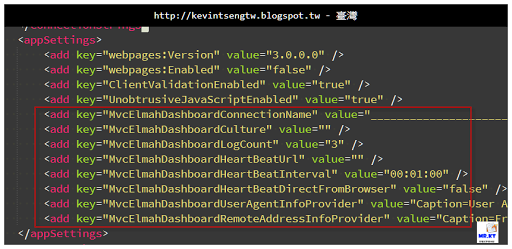MvcElmahDashboardConnectionName，這個要改為 Elmah 存放到資料庫的連線名稱

MvcElmahDashboardCulture，這個看各地區的語言文化來決定，例如可以填入 en-us or zh-tw，會影響日期時間的顯示格式

MvcElmahDashboardLogCount，這個是設定在 Dashboard 首頁裡要顯示多少則最新的紀錄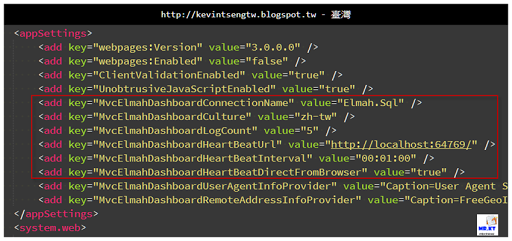Step.6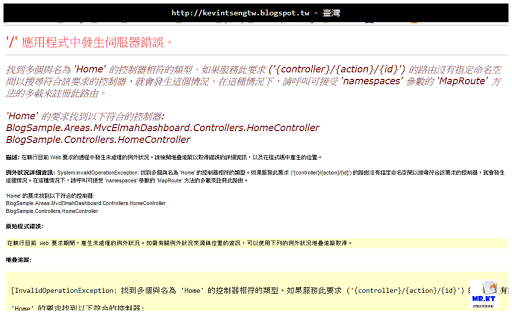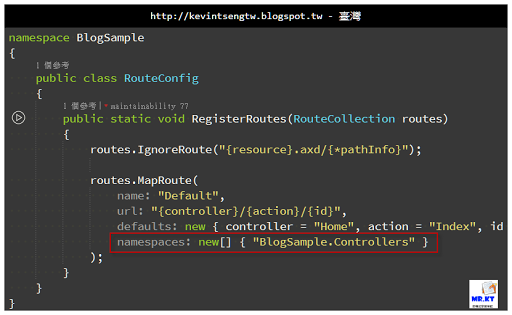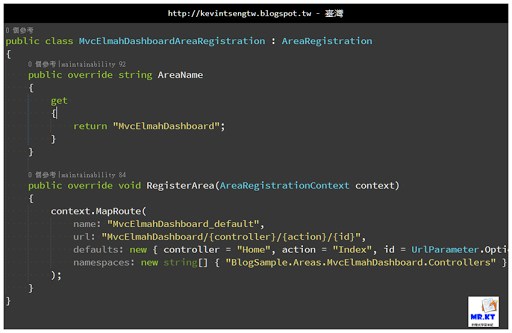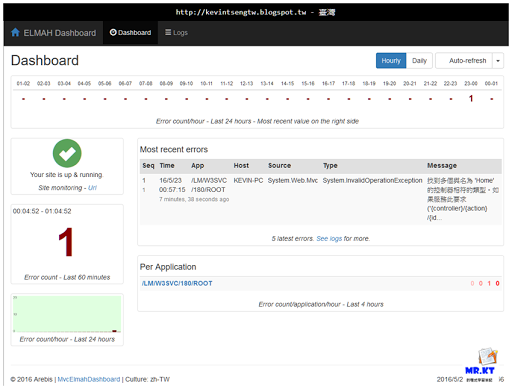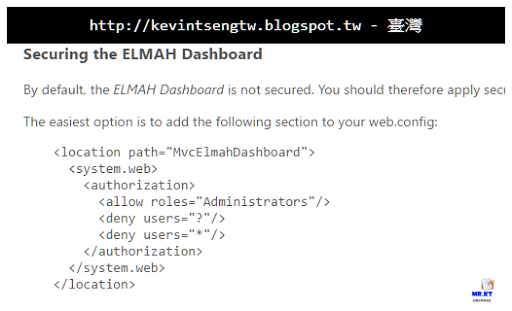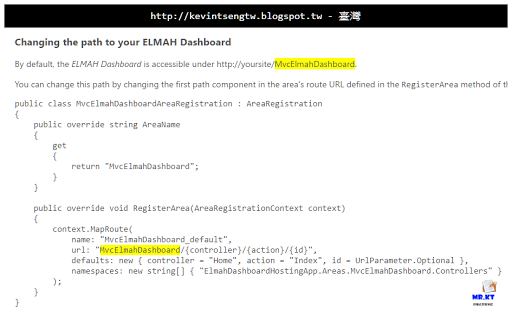### 參考連結

MVC ELMAH Dashboard
http://www.arebis.be/MvcElmahDashboard/

MVC ELMAH Dashboard Readme

NuGet Gallery| ASP.NET MVC ELMAH Dashboard 1.0.0
https://www.nuget.org/packages/Arebis.Web.Mvc.ElmahDashboard/

Configuring ELMAH to use SQL Server ~ andyfrench.info
http://www.andyfrench.info/2014/07/configuring-elmah-to-use-sql-server.html

### 延伸閱讀

mrkt 的程式學習筆記: 系統記錄與效能監測
http://kevintsengtw.blogspot.tw/p/blog-page_12.html

#### 2 則留言:

1.你好，照著教學在本機建了一個專案，發現用 IIS Express 跑錯誤，可以成功記錄到錯誤到 DB；但是如果改成 Local IIS 執行的便無法攔截錯誤記錄到 DB，是否可以請教這有可能是什麼原因造成的?

1.你所使用的 DB 是那一種呢？
LocalDB SqlExpress or 一般的 Sql Server ?
三種是有差異的，你可以先去了解這些 db 的不同和使用限制，你就會知道答案